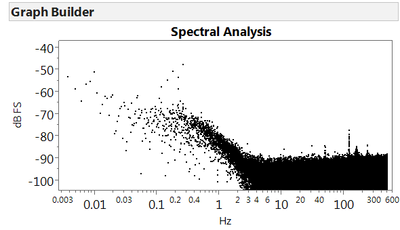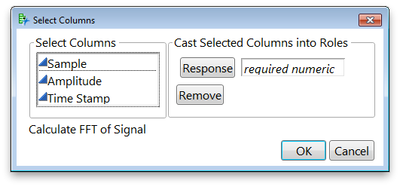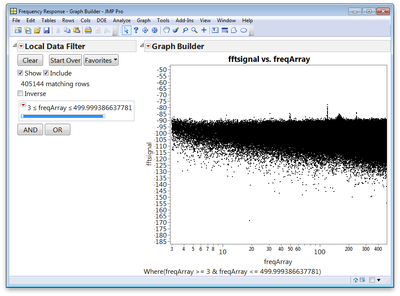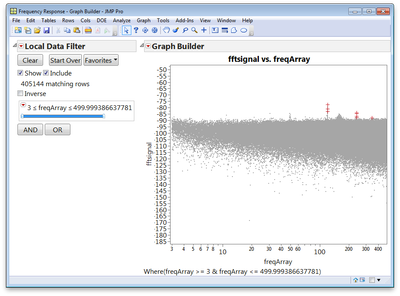It’s World Statistics Day! To honor the theme of the day, the JMP User Community is having conversations about the importance of trust in statistics and data. And we want to hear from you! Tell us the steps you take to ensure that your data is trustworthy.
Choose Language Hide Translation Bar
Highlighted

## How do I perform spectral analysis in JMP?

I've got a data series of over 800k points that I'd like to look at in the frequency domain.  I tried doing a "Time Series Analysis" to create spectral density data, but JMP just hangs.  I let it hang for several minutes, and then my graphics card died...  quite a coincidence!

The data file is attached if you want to try it out, first column is the time stamp in seconds, second column is the data.  Please let me know if there is a way to do this.

Thanks,

Jason

2 ACCEPTED SOLUTIONS

Accepted Solutions
Highlighted

## Re: How to do spectral analysis?

The Spectral Density command in Time Series is apparently using a slow algorithm. There is a JSL function called "FFT" that will use a fast algorithm, but only if the number of points is a nice round number (has lots of small factors). For instance, if I truncate your data to exactly 800,000 rows, the following JSL returns immediately:

data = Column( “y” ) << get values; Show( N Rows( data ) );

f = FFT( {data} ); Show( N Rows( f ) );

Current Data Table() << New Column("freq", Set Values( f ));

Highlighted

## Re: How to do spectral analysis?

Ran these data through an FFT analysis script I have written in MATLAB using the JMP interface to MATLAB in JMP 11. Looks like your SR is 1000Hz, so this gives you resolution up to your Nyquist freq. of 500 Hz. Lot's of LF energy towards DC, but there does seem to be some interesting peaks between 40 Hz - 300 Hz. Is this the type of result you might expect for these data?

Plotted in Graph Builder with the results returned from MATLAB:Check out the JMP blog: jmp.com/blog
6 REPLIES 6
Highlighted

## Re: How to do spectral analysis?

The Spectral Density command in Time Series is apparently using a slow algorithm. There is a JSL function called "FFT" that will use a fast algorithm, but only if the number of points is a nice round number (has lots of small factors). For instance, if I truncate your data to exactly 800,000 rows, the following JSL returns immediately:

data = Column( “y” ) << get values; Show( N Rows( data ) );

f = FFT( {data} ); Show( N Rows( f ) );

Current Data Table() << New Column("freq", Set Values( f ));

Highlighted

## Re: How to do spectral analysis?

Ran these data through an FFT analysis script I have written in MATLAB using the JMP interface to MATLAB in JMP 11. Looks like your SR is 1000Hz, so this gives you resolution up to your Nyquist freq. of 500 Hz. Lot's of LF energy towards DC, but there does seem to be some interesting peaks between 40 Hz - 300 Hz. Is this the type of result you might expect for these data?

Plotted in Graph Builder with the results returned from MATLAB:Check out the JMP blog: jmp.com/blog
Highlighted

## Re: How to do spectral analysis?

Thanks for looking at it!  Yes, I found some other software (Labchart, by AD Instruments) to do the FFT, and agree that there are a few interesting peaks.  The 120 Hz and 240 Hz are indicative of noise from a rectified AC source.  Some of the < 1 Hz energy resolves into peaks with a consistent multiple, possibly correlating to the rotational frequency of mechanical components in the system.

Mostly I posted to see if there was some functionality in JMP that I had not been using properly, but it appears that frequency domain statistical analysis is really not their thing.

Highlighted

## Re: How to do spectral analysis?

Excellent! Thanks for the info on those data. I am glad my analysis matches what you are expecting. JMP is actually very well suited for the statistical analysis of the frequency domain data, but some other tools (like MATLAB) are well-suited to do specialized things like perform FFTs on arbitrarily-sized arrays, filter designs, convolution etc. This is why the extensibility in JMP is great -- being able to reach out to SAS, MATLAB and R affords the ability to leverage these tools when needed and then bring the data back to JMP for visualization and analysis.

I ran your data through a FFT application I wrote all in JSL -- it works just like a JMP Platform:What it's doing when you click OK is connecting to MATLAB and running some preexisting code that converts the time-domain data to the frequency domain and then returns the data back to a new JMP data table for further analysis and graphing. If I needed to do additional processing of the signal in MATLAB -- say run a band-pass filter for example -- I could do that in MATLAB as well before returning the data to JMP.

But then when the data is back in JMP, I can analyze or visualize as I want. For example, I used a local data filter to just focus on the part of the signal where the rectified AC source components would be > 3 Hz.And then use Rows > Row Selection > Select Dominant to focus on the points on the Pareto Front. JMP identified 33 FFT bins, which I can subset using Tables > Subset. If I check the "linked to original data table" then the subset and the full data set will be linked. I put a + marker and colored these points in red in the subset, and they are are colored back in the original data table.Depending on what question you are trying to answer will dictate the best way to analyze these data, but once the transformed data is back into JMP, you can explore it any way you want. @Xan's suggestion to use the FFT function in JSL is great too. I just choose to use MATLAB because I already have a library of code that does a variety of signal processing things in MATLAB, and I also often need to filter data using IIR or FIR filters as part of the analysis step, a task that MATLAB is well-suited for (for both designing the filters, and performing the filtering step).

Check out the JMP blog: jmp.com/blog
Highlighted

## Re: How to do spectral analysis?

Could you share your script and let me try to run FFT in my data?

Highlighted

## Re: How to do spectral analysis?

Hello Daniel,

I second Fiona's request. Is it possible for you to share the code?

thanks

Article Labels

There are no labels assigned to this post.